# 基于双侧空间窗的异常检测方法Abnormal Detection Method Based on Bilateral Space Windows

• 全文下载: PDF(1669KB)    PP.19-27   DOI: 10.12677/CSA.2019.91003
• 下载量: 163  浏览量: 221

Confronting with the challenge of the difficult interpretation of the abnormal properties in previous works, a novel abnormal detection method based on bilateral space windows is proposed in our work. Firstly, after foreground construction, bilateral space windows are proposed to sample on the boundary of monitoring area, whose features can effectively describe the interesting regions. Secondly, in order to extract the attributions of speed, correlation and time delay, we design sequential cross-correlation measurement and analyze its theoretical and practical characteristics. Finally, we train our abnormal detection model using max margin framework, which considers both attributions of speed, correlation and time delay and additional appearance feature using fast Fourier transform. In the BEHAVE dataset with actual monitoring conditions, our method outperforms state-of-the-art methods both in AP and AUC evaluation. Moreover, even without prior, the method can automatically identify the location of the entrance and exit of the surveillance scene.

1. 引言

1) 通过提出的双侧空间窗特征，利用互相关分析方法，实现进入和离开的异常事件的自动判定。

2) 对场景中异常区域进行双侧空间窗采样，利用时序互相关分析，自动发现并定位监控场景中出入口的语境信息。

3) 根据监控任务的实际需求，将异常模式根据特性细分为：速度属性，出入属性和目标类属性，构建多属性分类器，实现不同人车不同类别目标的异常检测。

2. 时序互相关异常检测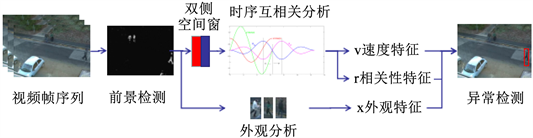Figure 1. Anomaly detection based on time series cross correlation analysis

2.1. 基于时序互相关的速度分析

${I}_{t}\left(x,y\right)=\left\{{I}_{h,t}\left(x,y\right),{I}_{s,t}\left(x,y\right),{I}_{i,t}\left(x,y\right)\right\}$ (1)

${F}_{t}\left(x,y\right)=\left\{\begin{array}{cc}1& {‖{I}_{t}\left(x,y\right)-{I}_{t-1}\left(x,y\right)‖}_{2}>\eta \cdot H\\ 0& {‖{I}_{t}{\left(x,y\right)}_{t}-{I}_{t-1}\left(x,y\right)‖}_{2}\le \eta \cdot H\end{array}$ (2)

${‖{I}_{t}\left(x,y\right)-{I}_{t-1}\left(x,y\right)‖}_{2}$ 为两帧像素的HSI向量的2范数， $\eta \cdot H$ 为帧差阈值，其取值方法为，首先估计视频帧中最大的帧差 $H={\mathrm{max}}_{x,y,t}{‖{I}_{t}\left(x,y\right)-{I}_{t-1}\left(x,y\right)‖}_{2}$ ，并将帧差与最大值比例大于 $\eta$ 的像素，认为是显著运动，本文方法中 $\eta$ 取值0.3。

${M}^{t}\left(D\right)={\sum }_{\left(x,y\right)\in D}{F}_{t}\left(x,y\right)$ (3)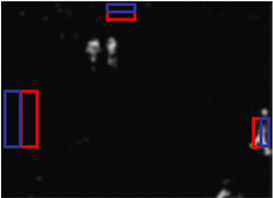(a)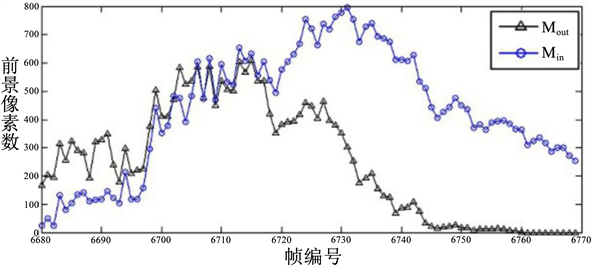(b)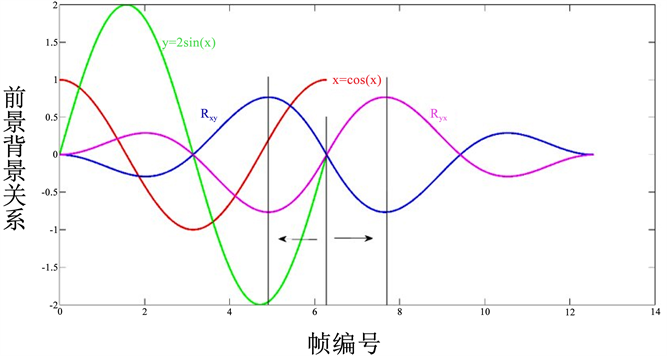(c)

Figure 2. The foreground pixel change of the bilateral space window when target entering the scene, (a) Bilateral space, (b) The foreground of the bilateral spatial window of the scene target changes, (c) Ideally bilateral spatial window correlation

${v}^{t}=\frac{1}{2\left({N}_{t}+1\right)}\underset{j=t}{\overset{t+{N}_{t}}{\sum }}\left({M}_{\text{in}}^{j}-{M}_{\text{in}}^{j-1}\right)+\left({M}_{\text{out}}^{j}-{M}_{\text{out}}^{j-1}\right)$ (4)

2.2. 基于时序互相关的时间差分析

$r{e}^{t}\left(\tau \right)=\frac{1}{{N}_{t}+1}\underset{j=t}{\overset{t+{N}_{t}}{\sum }}\frac{{M}_{\text{in}}^{j}}{|{D}_{\text{in}}|}\frac{{M}_{\text{out}}^{j+\tau }}{|{D}_{\text{out}}|}$ (5)

${r}^{t},{\tau }^{t}={\mathrm{max}}_{r,\tau }r{e}^{t}\left(\tau \right)$ (6)

2.3. 联合时序互相关和外观特征的异常检测

$\Omega \left(w\right)=\frac{1}{2}{‖w‖}^{2}+〈{w}^{T}\cdot z〉$ (7)

3. 实验

3.1. 场景出入口语境发现Figure 3. Scene entry and exit position detection based on bilateral space windowTable 1. Entrance and exit detection and correlation analysis in monitoring scene

3.2. 异常检测Table 2. Evaluation of abnormal monitoring of BEHAVE database in real monitoring scene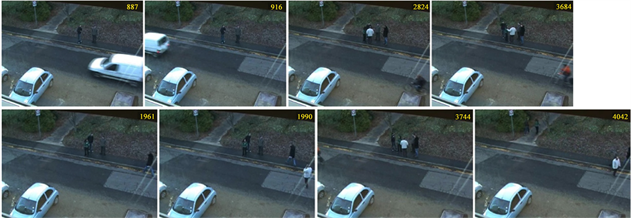Figure 4. Anomaly detection results of BEHAVE database in real monitoring scene, the first line: transportation (car, bicycle); the second line: pedestrians

4. 结论

  Zhang, T., Jia, W., Gong, C., et al. (2017) Semi-Supervised Dictionary Learning via Local Sparse Constraints for Vio-lence Detection. Pattern Recognition Letters, 1-8.  Cong, Y., Yuan, J. and Liu, J. (2013) Abnormal Event Detection in Crowded Scenes Using Sparse Representation. Pattern Recognition, 46, 1851-1864. https://doi.org/10.1016/j.patcog.2012.11.021  Hassner, T., Itcher, Y. and Kliper-Gross, O. (2012) Violent Flows: Real-Time Detection of Violent Crowd Behavior. IEEE Computer Society Conference on Computer Vision and Pattern Recognition Workshops (CVPRW), Providence, RI, 16-21 June 2012, 1-6.  Nievas, E.B., Suarez, O.D., García, G.B., et al. (2011) Violence Detection in Video Using Computer Vision Techniques. International Conference on Computer Analysis of Images and Patterns, Springer, Berlin, Heidelberg, 332-339.  Zhang, T., Jia, W., Yang, B., et al. (2017) Mowld: A Robust Motion Image Descriptor for Violence Detection. Multimedia Tools and Applications, 76, 1419-1438. https://doi.org/10.1007/s11042-015-3133-0  Li, W., Mahadevan, V. and Vasconcelos, N. (2014) Anomaly Detection and Localization in Crowded Scenes. IEEE Transactions on Pattern Analysis and Machine Intelli-gence, 36, 18-32. https://doi.org/10.1109/TPAMI.2013.111  Hu, W., Xiao, X., Fu, Z., et al. (2006) A System for Learning Statistical Motion Patterns. IEEE Transactions on Pattern Analysis and Machine Intelligence, 28, 1450-1464. https://doi.org/10.1109/TPAMI.2006.176  Mehran, R., Oyama, A. and Shah, M. (2009) Abnor-mal Crowd Behavior Detection Using Social Force Model. IEEE Conference on Computer Vision and Pattern Recogni-tion, CVPR 2009, Miami, FL, 20-25 June 2009, 935-942.  Wang, D., Zhang, X., Fan, M., et al. (2016) Semi-Supervised Dictionary Learning via Structural Sparse Preserving. AAAI, 2137-2144.  Babagholami-Mohamadabadi, B., Zarghami, A., Zolfaghari, M., et al. (2013) Pssdl: Probabilistic Semi-Supervised Dictionary Learning. Joint European Conference on Machine Learning and Knowledge Discovery in Databases, Springer, Berlin, Heidelberg, 192-207.  Wright, J., Yang, A.Y., Ganesh, A., et al. (2009) Robust Face Recognition via Sparse Representation. IEEE Transactions on Pattern Analysis and Machine Intelligence, 31, 210-227. https://doi.org/10.1109/TPAMI.2008.79  蔡瑞初, 谢伟浩, 郝志峰, 等. 基于多尺度时间递归神经网络的人群异常检测[J]. 软件学报, 2015, 26(11): 2884-2896.  Xu, D., Yan, Y., Ricci, E., et al. (2017) Detecting Anom-alous Events in Videos by Learning Deep Representations of Appearance and Motion. Computer Vision and Image Un-derstanding, 156, 117-127. https://doi.org/10.1016/j.cviu.2016.10.010  Henriques, J.F., Carreira, J., Caseiro, R., et al. (2013) Beyond Hard Negative Mining: Efficient Detector Learning via Block-Circulant Decomposition. IEEE International Conference on Computer Vision (ICCV), Sydney, 1-8 December 2013, 2760-2767.